# Using Multiplication to Cut Gates from the QFT

14 Jun 2016

In this post: pulling a multiplication out of the phase corrections performed during a quantum Fourier transform, and using it to save gates.

# Fourier Construction

Here's a typical circuit for the Quantum Fourier Transform: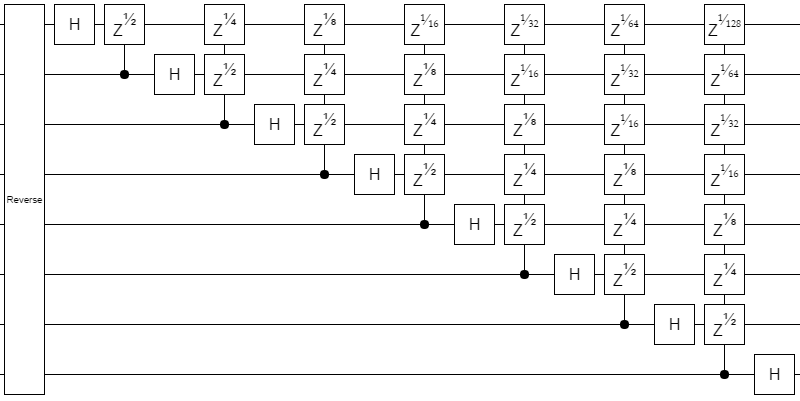It's basically just the Cooley-Tukey FFT algorithm translated directly into a quantum circuit. The Hadamards are the sums-and-differences part, the Zs are the phase gradient part, and the interleaving/recursing part is implicit in the positioning of the gates and the bit reversal.

One way to make the circuit look nicer is to separate the Hadamard gates from the phase gates. There's two ways to do that: we can push the Hadamards to the right, or to the left.

If we push the Hadamards to the right, they cross over the Z gates. This turns the Z gates into X gates: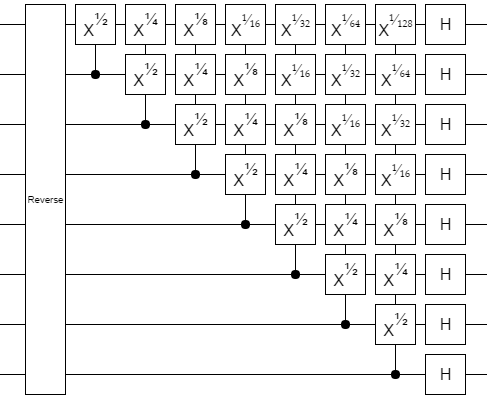If we push the Hadamards to the left, they cross over the Z-is-On controls and turn them into X-is-On controls: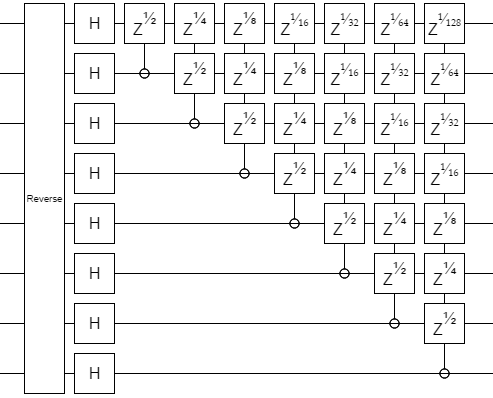Note that the above circuit is going through all the qubit pairs, and applying phases based on the AND of the pair's first qubit's Z-value and the second qubit's X value. Also note that if you look at the shape formed by each type of gate, you get diagonals.

To me, those diagonals-of-ways-you-can-combine-two-values scream convolution because my mental image of convolution is summing up the diagonals of a grid: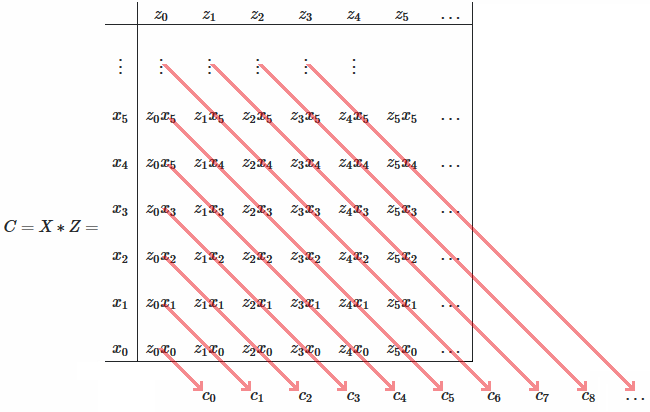Actually, because each diagonal corresponds to how many times a phase gate that's twice as powerful as the last is applied, we also have a carrying effect: 2 of a diagonal is worth 1 of the next diagonal. So this isn't convolution, it's multiplication. We're multiplying the litte-endian X-value of a block of qubits by the Z-value of those same qubits, and phasing based on the result!

Okay, that's not quite right. The uncertainty principle prevents qubits from having an X-value and a Z-value at the same time. But we can pull out quite a large block where we only use one of the values for each qubit: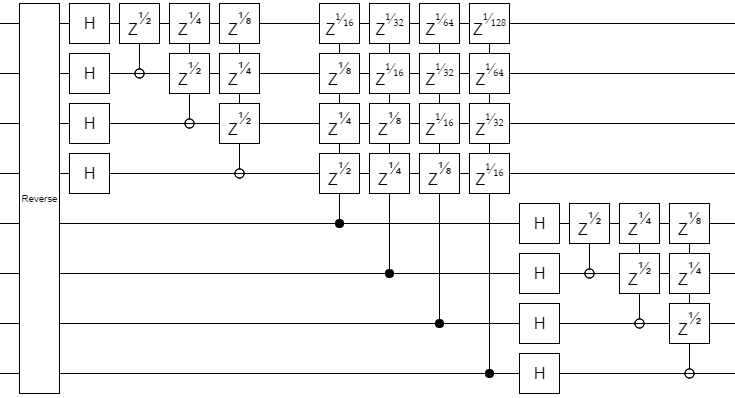And then we can replace that block of controlled phase gradients with a multiplication and a single phase gradient: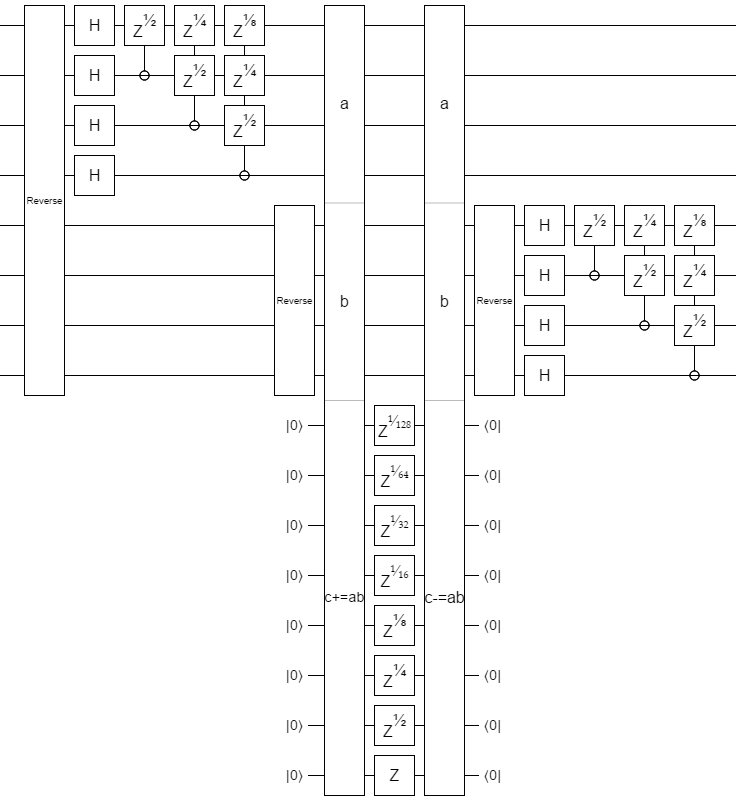In terms of asymptotic gate count, this is an optimization. We replaced $O(n^2)$ gates with a construction that uses $O(M(n) + n)$ gates, where $M(n)$ is the number of gates needed to perform a multiplication. It's known that $M(n) \in O(n (\lg n) (\lg \lg n))$, and conjectured that $M(n) \in O(n \lg n)$, so this is an improvement if $n$ is large enough.

Also notice that the parts before and after the multiplication are basically just half-sized QFTs. With some slight tweaks, we find this construction: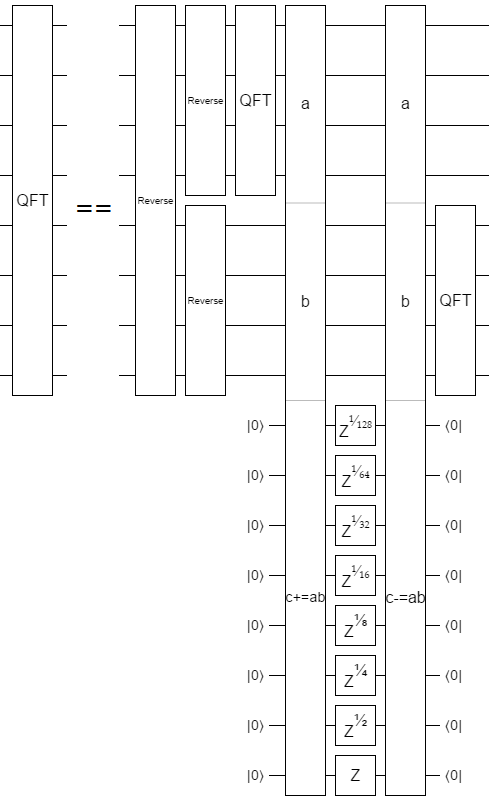Which has a gate-count recurrence relation of $F(n) = 2 F(n/2) + M(n)$. Solve that out, and you find that $F(n) \in O(\lg(n) M(n))$.

Therefore this construction uses at most $O(n (\lg^2 n) (\lg \lg n))$ gates, and possibly $O(n \lg^2 n)$ gates. That's a pretty large improvement from the original construction's $O(n^2)$! (Though in practice we might care more about depth than count, not to mention topological constraints.)

As with most things I stumble onto on my own, this is not an original discovery. See the paper Fast parallel circuits for the quantum Fourier transform by Richard Cleve and John Watrous from Jan 2000.

# Classical Uses?

You might expect that, since we started with a direct translation of the Cooley-Tukey algorithm and then asymptotically improved it, the new construction can be translated back for savings in the classical case. Unfortunately, that's not correct. It comes down to the fact that the phase gradient after each Hadamard could already be applied in a single sweep. We'd just be replacing that simple sweep with a more complicated sweep.

(However it is interesting that the complicated sweeps are slightly more biased away from fine-grained twiddle factors.)

(Ironically, fast multiplication algorithms are themselves all based on Fourier transforms. We're using the classical Fourier transform to speed up the quantum Fourier transform.)

# Summary

The phasing part of the QFT kind of involves multiplying the little-endian Z-value of some qubits by their X-value. By extracting the multiplication, you can perform the QFT with asymptotically fewer gates than the naive construction.